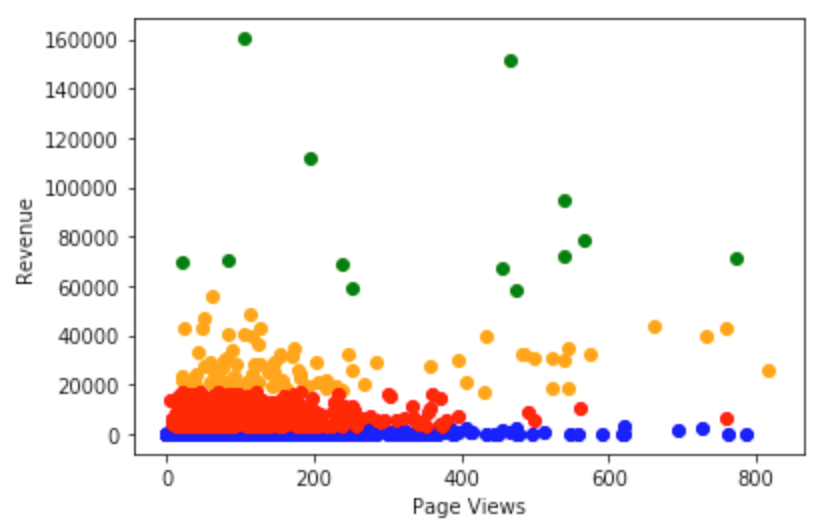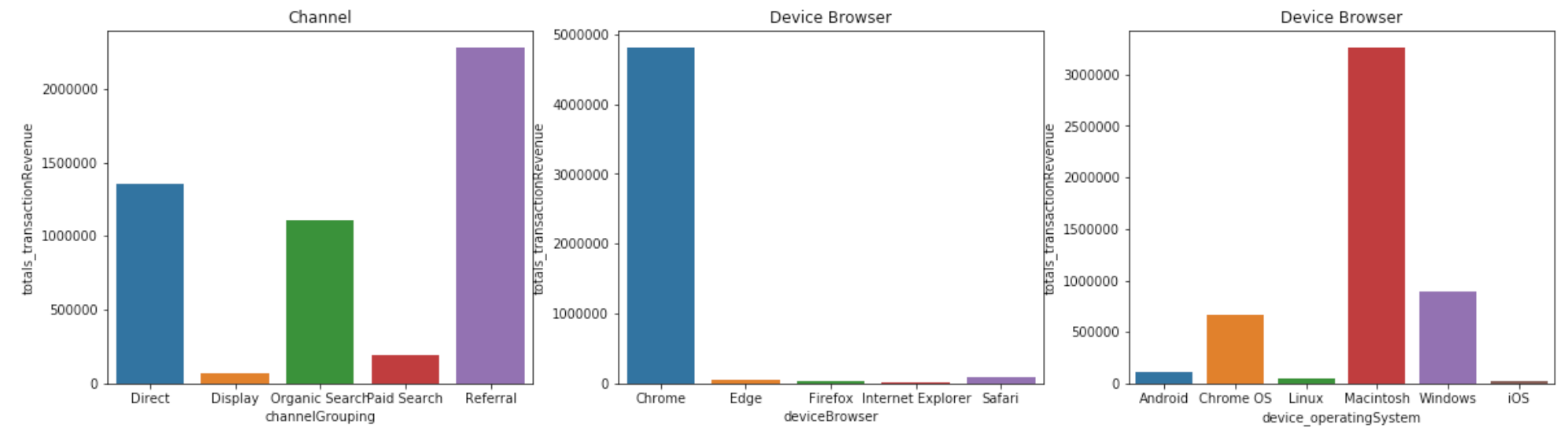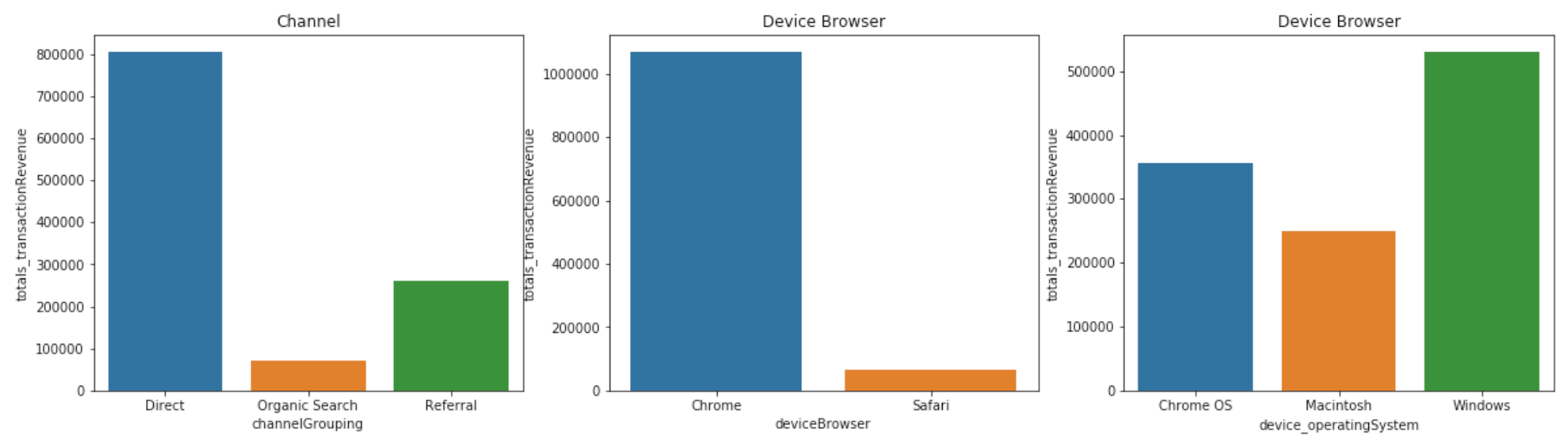# Customer Segmentation: K-Means Clustering & A/B TestingThis post was originally published by Andrew Nguyen at Towards Data Science

### Context

I have been working in Advertising, specifically Digital Media and Performance, for nearly 3 years and customer behaviour analysis is one of the core concentrations in my day-to-day job. With the help of different analytics platforms (e.g. Google Analytics, Adobe Analytics), my life has been made easier than before since these platforms come with the built-in function of segmentation that analyses user behaviours across dimensions and metrics.

However, despite the convenience provided, I was hoping to leverage Machine Learning to do customer segmentation that can be scalable and applicable to other optimizations in Data Science (e.g. A/B Testing). Then, I came across the dataset provided by Google Analytics for a Kaggle competition and decided to use it for this project.

Feel free to check out the dataset here if you’re keen! Beware that the dataset has several sub-datasets and each has more than 900k rows!

### A. Explanatory Data Analysis (EDA)

This always remain an essential step in every Data Science project to ensure the dataset is clean and properly pre-processed to be used for modelling.

First of all, let’s import all the necessary libraries and read the csv file:

```import pandas as pd
import matplotlib.pyplot as plt
import numpy as np

### 1. Flatten JSON Fields

As you can see, the raw dataset above is a bit “messy” and not digestible at all since some variables are formatted as JSON fields which compress different values of different sub-variables into one field. For example, for geoNetwork variable, we can tell that there are several sub-variables such as continent, subContinent, etc. that are grouped together.

Thanks to the help of a Kaggler, I was able to convert these variables to a more digestible ones by flattening those JSON fields:

```import os
import json
json_columns = ['device', 'geoNetwork', 'totals', 'trafficSource']
for column in json_columns:
column_converted = json_normalize(df[column])
column_converted.columns = [f"{column}_{subcolumn}" for subcolumn in column_converted.columns]
df = df.drop(column, axis=1).merge(column_converted, right_index=True, left_index=True)
return df```

After flattening those JSON fields, we are able to see a much cleaner dataset, especially those JSON variables split into sub-variables (e.g. device split into device_browser, device_browserVersion, etc.).

### 2. Data Re-formatting & Grouping

For this project, I have chosen the variables that I believe have better impact or correlation to the user behaviours:

```df = df.loc[:,['channelGrouping', 'date', 'fullVisitorId', 'sessionId', 'visitId', 'visitNumber', 'device_browser', 'device_operatingSystem', 'device_isMobile', 'geoNetwork_country', 'trafficSource_source', 'totals_visits', 'totals_hits', 'totals_pageviews', 'totals_bounces', 'totals_transactionRevenue']]df = df.fillna(value=0)

Moving on, as the new dataset has fewer variables which, however, vary in terms of data type, I took some time to analyze each and every variable to ensure the data is “clean enough” prior to modelling. Below are some quick examples of un-clean data to be cleaned:

```#Format the values
df.channelGrouping.unique()
df.channelGrouping = df.channelGrouping.replace("(Other)", "Others")#Convert boolean type to string
df.device_isMobile.unique()
df.device_isMobile = df.device_isMobile.astype(str)
df.loc[df.device_isMobile == "False", "device"] = "Desktop"
df.traffic_source[df.traffic_source.str.contains("baidu")] = "baidu"
df.traffic_source[df.traffic_source.str.contains("bing")] = "bing"
df.traffic_source[df.traffic_source.str.contains("yahoo")] = "yahoo"
.....
df.traffic_source[~df.traffic_source.isin(main_traffic_source)] = "Others"```

After re-formatting, I found that fullVisitorID’s unique values are fewer than the total rows of the dataset, meaning there are multiple fullVisitorIDs that were recorded. Hence, I proceeded to group the variables by fullVisitorID and sort by Revenue:

```df_groupby = df.groupby(['fullVisitorId', 'channelGrouping', 'geoNetwork_country', 'traffic_source', 'device', 'deviceBrowser', 'device_operatingSystem'])
.agg({'totals_hits':'sum', 'totals_pageviews':'sum', 'totals_bounces':'sum','totals_transactionRevenue':'sum'})
.reset_index()df_groupby = df_groupby.sort_values(by='totals_transactionRevenue', ascending=False).reset_index(drop=True)```

### 3. Outlier Handling

The last step of any EDA process that cannot be overlooked is detecting and handling outliers of the dataset. The reason being is that outliers, especially those marginally extreme ones, impact the performance of a machine learning model, mostly negatively. That said, we need to either remove those outliers from the dataset or convert them (by mean or mode) to fit them to the range that the majority of the data points lie in:

```#Seaborn Boxplot to see how far outliers lie compared to the rest
sns.boxplot(df_groupby.totals_transactionRevenue)```

As you can see, most of the data points in Revenue lie below USD200,000 and there’s only one extreme outlier that hits nearly USD600,000. If we don’t remove this outlier, the model also takes it into consideration that produces a less objective reflection.

So let’s go ahead and remove it, and please do so for other variables. Just a quick note, there are several methods of dealing with outliers (such as inter-quantiles). However, in my case, there’s only one so I just went ahead defining the range that I believe fits well:

`df_groupby = df_groupby.loc[df_groupby.totals_transactionRevenue < 200000]`

### B. K-Means Clustering

What is K-Means Clustering and how does it help with customer segmentation?

Clustering is the most well-known unsupervised learning technique that finds structure in unlabeled data by identifying similar groups/clusters, particularly with the helps of K-Means.

K-Means tries to address two questions: (1) K: the number of clusters (groups) we expect to find in the dataset and (2) Means: the average distance of data to each cluster center (centroid) which we try to minimize.

Also, one thing of note is that K-Means comes with several variations, typically :

1. init = ‘random’: that randomly selects the centroids of each cluster
2. init = ‘k-means++’: that only selects the 1st centroid by randomness while other centroids to be placed as far away from the 1st as possible

In this project, I’ll use the second option to ensure that each cluster is well-distinguished from one another:

```from sklearn.cluster import KMeansdata = df_groupby.iloc[:, 7:]kmeans = KMeans(n_clusters=3, init="k-means++")
kmeans.fit(data)labels = kmeans.predict(data)
labels = pd.DataFrame(data=labels, index = df_groupby.index, columns=["labels"])```

Before applying the algorithm, we need to define “n_clusters” which is the number of groups we expect to get out of the modelling. In this case, I randomly put n_clusters = 3. Then, I went ahead visualizing how the dataset is grouped using 2 variables: Revenue and PageViews:

`plt.scatter(df_kmeans.totals_transactionRevenue[df_kmeans.labels == 0],df_kmeans.totals_pageviews[df_kmeans.labels == 0], c='blue')plt.scatter(df_kmeans.totals_transactionRevenue[df_kmeans.labels == 1], df_kmeans.totals_pageviews[df_kmeans.labels == 1], c='green')plt.scatter(df_kmeans.totals_transactionRevenue[df_kmeans.labels == 2], df_kmeans.totals_pageviews[df_kmeans.labels == 2], c='orange')plt.show()`

As you can see, the x-axis stands for the number of Revenue while y-axis for PageViews . After modelling, we can tell a certain degree of difference in 3 clusters. However, I was not sure whether 3 is the “right” number of clusters or not. That said, we can rely on the estimator of K-Means algorithm, inertia_, which is the distance from each sample to the centroid. In particular, we will compare the inertia of each cluster ranging from 1 to 10, in my case, and see which is the lowest and how far we should go:

```#Find the best number of clustersnum_clusters = [x for x in range(1,10)]
inertia = []for i in num_clusters:
model = KMeans(n_clusters = i, init="k-means++")
model.fit(data)
inertia.append(model.inertia_)

plt.plot(num_clusters, inertia)
plt.show()```

From the chart above, inertia started to fall slowly since the 4th or 5th cluster, meaning that that’s the lowest inertia we can get, so I decided to go with “n_clusters=4”:

```plt.scatter(df_kmeans_n4.totals_pageviews[df_kmeans_n4.labels == 0], df_kmeans_n4.totals_transactionRevenue[df_kmeans_n4.labels == 0], c='blue')plt.scatter(df_kmeans_n4.totals_pageviews[df_kmeans_n4.labels == 1],
df_kmeans_n4.totals_transactionRevenue[df_kmeans_n4.labels == 1], c='green')plt.scatter(df_kmeans_n4.totals_pageviews[df_kmeans_n4.labels == 2],
df_kmeans_n4.totals_transactionRevenue[df_kmeans_n4.labels == 2], c='orange')plt.scatter(df_kmeans_n4.totals_pageviews[df_kmeans_n4.labels == 3],
df_kmeans_n4.totals_transactionRevenue[df_kmeans_n4.labels == 3], c='red')plt.xlabel("Page Views")
plt.ylabel("Revenue")plt.show()```Switch PageViews to x-axis and Revenue to y-axis

The clusters now look a lot more distinguishable from one another:

1. Cluster 0 (Blue): high PageViews yet little-to-none Revenue
2. Cluster 1 (Red): medium PageViews, low Revenue
3. Cluster 2 (Orange): medium PageViews, medium Revenue
4. Cluster 4 (Green): unclear trend of PageViews, high Revenue

Except for cluster 0 and 4 (unclear pattern), which are beyond our control, cluster 1 and 2 can tell a story here as they seem to share some similarities.

To understand which factor that might impact each cluster, I segmented each cluster by Channels, Device and Operating System:Cluster 1Cluster 2

As seen from above, in Cluster 1, Referral channel contributed the highest Revenue, followed by Direct and Organic Search. In contrast, it’s Direct that made the highest contribution in Cluster 2. Similarly, while Macintosh is the most dominating device in Cluster 1, it’s Windows in Cluster 2 that achieved higher revenue. The only similarity between 2 clusters is the Device Browser, which Chrome is widely used.

Voila! This further segmentation helps us tell which factor (in this case, Channel, Device Browser, Operating System) works better for each cluster, hence we can better evaluate our investment moving forward!

### C. A/B Testing through Hypothesis Testing

What is A/B Testing and how can Hypothesis Testing come into place to complement the process?

A/B Testing is no stranger to those who work in Advertising and Media, since it’s one of the powerful techniques that help improve the performance with more cost efficiency. Particularly, A/B Testing divides the audience into 2 groups: Test vs Control. Then, we expose the ads/show a different design to the Test group only to see if there’s any significant discrepancy between 2 groups: exposed vs un-exposed.

In Advertising, there are a number of different automatic tools in the market that can easily help do A/B Testing at one click. However, I still wanted to try a different method in Data Science that can do the same: Hypothesis Testing. The methodology is pretty much the same, as Hypothesis Testing compares the Null Hypothesis (H0) and Alternate Hypothesis (H1) and see if there’s any significant discrepancy between the two!

Assume that I run a promotion campaign that exposes an ad to the Test group. Here’s a quick summary of steps that need to be followed to test the result with Hypothesis Testing:

1. Sample Size Determination
2. Pre-requisite Requirements: Normality and Correlation Tests
3. Hypothesis Testing

For the 1st step, we can rely on Power Analysis which helps determine the sample size to draw from a population. Power Analysis requires 3 parameters: (1) effect size, (2) power and (3) alpha. If you are looking for details on how Power Analysis, please refer to an in-depth article here that I wrote some time ago.

Below is a quick note to each parameter for your quick understanding:

```#Effect Size: (expected mean - actual mean) / actual_std
effect_size = (280000 - df_group1_ab.revenue.mean())/df_group1_ab.revenue.std() #set expected mean to \$350,000
print(effect_size)#Power
power = 0.9 #the probability of rejecting the null hypothesis#Alpha
alpha = 0.05 #the error rate```

After having 3 parameters ready, we use TTestPower() to determine the sample size:

`import statsmodels.stats.power as smsn = sms.TTestPower().solve_power(effect_size=effect_size, power=power, alpha=alpha)print(n)`

The result is 279, meaning we need to draw 279 data points from each group: Test and Control. As I don’t have real data, I used np.random.normal to generate a list of revenue data, in this case sample size = 279 for each group:

```#Take the samples out of each group: control vs testcontrol_sample = np.random.normal(control_rev.mean(), control_rev.std(), size=279)
test_sample = np.random.normal(test_rev.mean(), test_rev.std(), size=279)```

Moving to the 2nd step, we need to ensure the samples are (1) normally distributed and (2) independent (not correlated). Again, if you want a refresh on the tests used in this step, refer to my article as above. In short, we are going to use (1) Shapiro as the normality test and (2) Pearson as the correlation test.

```#Step 2. Pre-requisite: Normality, Correlationfrom scipy.stats import shapiro, pearsonrstat1, p1 = shapiro(control_sample)
stat2, p2 = shapiro(test_sample)print(p1, p2)stat3, p3 = pearsonr(control_sample, test_sample)
print(p3)```

The p-value of Shapiro is 0.129 and 0.539 for Control and Test group respectively, which is > 0.05. Hence, we don’t reject the null hypothesis and are able to say that 2 groups are normally distributed.

The p-value of Pearson is 0.98, which is >0.05, meaning that 2 groups are independent from each other.

Final step is here! As there are 2 variables to be tested against each other (Test vs Control group), we use T-Test to see if there’s any significant discrepancy in Revenue after running A/B Testing:

```#Step 3. Hypothesis Testingfrom scipy.stats import ttest_indtstat, p4 = ttest_ind(control_sample, test_sample)
print(p4)```

The result is 0.35, which is > 0.05. Hence, the A/B Test conducted indicates that the Test Group exposed to the ads doesn’t show any superiority over the Control Group with no ad exposure.

Voila! That’s the end of this project — Customer Segmentation & A/B Testing! I hope you find this article useful and easy to follow.

Do look out for my upcoming projects in Data Science and Machine Learning in the near future! In the meantime feel free to check out my Github here for the complete repository:

Thanks!